Next: Projection operators Up: Foundations Previous: Expansion in terms delta

# Bra-ket notation

(Read pgs. 109-144) The Dirac Bra-Ket notation is a concise and convenient way to describe quantum states. We introduce and define the symbol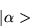(1.24)

to represent a quantum state. This is called a ket, or a ket vector. It is an abstract entity, and serves to describe the "state" of the quantum system. We say that a physical system is in quantum state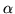, whererepresents some physical quantity, such as momentum, spin etc, when represented by the ket.

If we have two distinct quantum states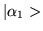and, then the following ket(1.25)

where ci is a complex number, is also a possible state for the system.

In general, the number of linear independent kets required to express any other ket, is called the dimension of the vector space. In quantum mechanics the vector space of kets is usually non denumerable infinite. We call such a vector space Hilbert space. We assume that any physical state can be described by a ket in Hilbert space.

Dirac defined something called a bra vector, designated by. This is not a ket, and does not belong in ket space e.g.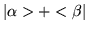has no meaning. However, we assume for every ket, there exists a bra labeled. The brais said to be the dual of the ket. We can ask the question: since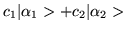is a ket, what is the dual (or bra vector) associated with that vector?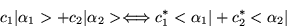(1.26)

where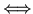signifies a dual correspondence. This is an anti-linear relation.

Dirac allowed the the bra's and ket's to line up back to back, i.e.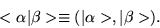(1.27)

The symbol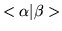represents a complex number that is equal to the value of the inner product of the ketwith. We note, according to the above definition, that,(1.28)

Dirac also defined something called an outer product,(1.29)

An outer product is allowed to stand next to a ket on its left, or next to a bra on the bra's right. Lets define, then if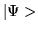is an arbitrary ket, one is allowed to construct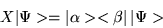(1.30)

It looks like we have something like an inner product on the r.h.s of this equation. Indeed, according to the associative axiom of multiplication, we are allowed to put parenthesis around the quantity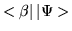and equate it to the value of the inner product. Or(1.31)

The outer product X is an operator in Hilbert space. It acts on ketfrom the left and turns it into another ket. Be careful! forhas no meaning, however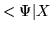does.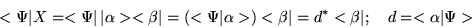(1.32)

If we take operator A and operate on a ket, is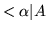dual to it? In general it is not, however the dual ofis(1.33)

whereis called the hermitian conjugate of operator A. Show that the hermitian conjugate ofis.

Sometimes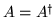, then A is called an hermitian operator. Hermitian operators play a central role in quantum theory. Show that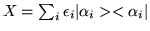, whereis a real number, is hermitian. Consider a hermitian operator X, whose eigenstates | a> obey the eigenvalue equation(1.34)

where a is an eigenvalue. Suppose these eigenvalues are distinct, then setare mutually orthonormal and form a set of basis kets in Hilbert space, provided that(1.35)

With this set we can express any ketby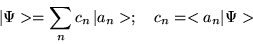(1.36)Next: Projection operators Up: Foundations Previous: Expansion in terms delta
Bernard Zygelman
1999-09-21